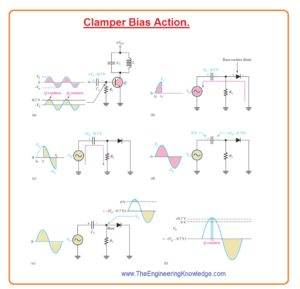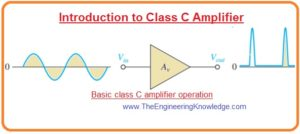Hello friends, I hope you all are doing great. In today’s tutorial, we will have a look at Introduction to Class C Amplifier. The Class C amplifier Category of amplifier in which transistor operates for a one-eighty degree of the input voltage signal. Normally its operate for eight to one twenty-degree of a signal. This operation for less signal increases the efficiency of this amplifier but creates some distortion. In theory, the highest efficiency of class C amplifier is almost ninety degrees.

Due to large signal distortion, this amplifier type is not good for acoustic applications. It mostly used in radio frequency circuitry such as oscillator, radio frequency amplifiers. The efficiency of the class C amplifier is larger than the class A, class B and class AB push-pull amplifiers. It means that power obtained at output is larger than other amplifiers. It not used for linear application circuits. In today’s post, we will have a detailed look at its working, circuit arrangements and some related parameters. So let’s get started with Introduction to Class C Amplifier.

#### Introduction to Class C Amplifier

Class C working operation

• The main operation of class C amplifier is explained in below figure.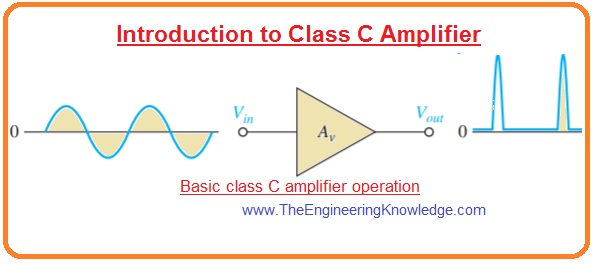• In the below figure the common emitter class C amplifier consisting of a load as resistance is shown in the below figure denoted as ‘a’.
• The class C amplifier usually functioned with a resonant circuitry load due to that resistance as load is used to discuss the main idea.
• This circuitry is biased with the negative power source denoted as VBB less than cutoff region.
• The peak value of ac voltage source is larger than the ΙVBBΙ+VBE so base voltage crosses the potential barrier value of base-emitter junction for less time interval close to the positive peak of every cycle is shown in figure denoted as ‘b’.
• For this duration, the transistor is off. If the complete ac load line is used as shown in figure denoted as ‘c’ the ideal extreme collector current will be Ic(sat) and ideal least collector voltage will be Vce(sat).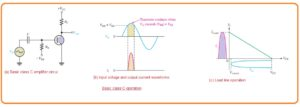#### Class C Amplifier Power Dissipation

• The power dissipation in class C amplifier is less since as we discussed above that it operates only some part of input waveform.
• In figure denoted as a pulses of collector current is shown.• The time among the pulses T of ac input signal. For on-time of transistor collector current and the collector, voltage is shown in figure denoted as ‘b’.
• If the output is moved above the complete load the max current amplitude will be
Ic(sat) and the min voltage amplitude is Vce(sat) for on-time of transistor.
• The power dissipation for on time of transistor will be.

PD(on) = Ic (sat)Vce (sat)

• The transistor is operating or on for ton remains off for the remaining time interval.
• By supposing that complete load line is used the average power dissipation for complete signal wave will be.

PD(avg)=ton/T(PD(on))

=(ton/T)Ic(sat)Vce (sat)

#### Class C Amplifier Tuned Operation

• As the output or collector voltage is not replica to the input voltage then class c amplifier connected with resistance as the load is alone if there is no value in linear application exits.
• So it is compulsory to use class c amplifier with the resonant circuit or tank circuit in parallel combination as shown in figure denoted as ‘a’.
• The resonant frequency of resonant circuit can be calculated by the formula fr=1/(2Π√LC).
• The small-signal pulse of the collector current on every wave cycle of input starts and retains the oscillations of the resonant circuit due to that output voltage is generated as shown in figure denoted as b.
• The impedance of resonant or tank circuitry is large near to the resonant frequency due to that gain is high only at this frequency value.• The pulses of current charges the capacitor almost value equal to VCC as shown in below figure denoted as ‘a’.
• After the movement of the pulse capacitor gets discharged rapidly and charges the inductor.
• When capacitor becomes completely discharged the magnetic field of inductor get collapse and then rapidly charge the capacitor again to the value of VCC in a direction which it firstly get charged.
• It completes the one halve signal cycle of oscillation as shown in figures denoted as b’ and c.
• After that transistor gets discharged again and increases the magnetic field of inductor.
• Then again inductor recharge the capacitor to positive peak value less then previous value it occurs due to loss of energy in the winding.
• In this way, it completes one cycle as shown in figure denoted as d and e.
• So the value of peak to peak output voltage will be almost 2VCC.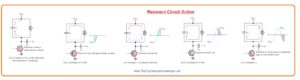• The amplitude of every succeeding cycle of the fluctuation will be less than the preceding cycle due to loss of energy in the resistance of tank circuitry as shown in figure denoted as ‘a’ and the oscillation will finally decease
• Nevertheless, the consistent repetitions of the collector current pulse re-energizes the tank circuitry and withstand the oscillations at a non-varying amplitude.
• When the resonant circuitry is adjusted to the frequency of input signal, the reenergize process happens on alternative cycles as shown in figure denoted as ‘c’.
• In this condition class C amplifier function lie frequency multiplier.#### Clamper Bias for a Class C Amplifier

• In below figure you can see the class C amplifier with base bias clamping configuration.
• The base-emitter junction operates as diode.• If the input voltage signal is positive capacitor C1 get charged to the peak value having polarity shown in below figure denoted as ‘a’.
• This process generates an average voltage at the base terminal almost -Vp.
• Due to it transistor is in cutoff region by letting the positive peaks when the transistor is operating for a small time interval.
• For a good clamping process, the time constant R1C1 of clamping circuitry should be larger than the time period of the input signal.
• The circuit denoted as b,c,d,e,f explains the bias clamping process.
• For the time up to the positive peak of input from t0 to t1 the capacitor to the value Vp-0.7volts through the base-emitter diode as denoted in figure ‘b’.
• For the time interval t1 to t2 as shown in figure c the capacitor get discharge very less due to large RC time constant.
• The capacitor so stores an average charge less than  Vp-0.7volts.
• As the dc value of input voltage is 0 at the positive side of capacitor c1 the value of dc voltage base is greater than the -(Vp-0.7) as shown in figure denoted as d.
• In figure ‘e’ the capacitor is linked with the base through the input voltage signal so the voltage at the base of the transistor is ac signal moving on the dc level largely positive value then
• so that the voltage at the transistor’s base is the ac signal riding on a dc level slightly more positive than-(Vp-0.7) .
• Close to the positive peak value of input voltage base voltage move to the somewhat over 0.7 volts and due to that transistor operate for a small time interval. It is shown in figure ‘f’.